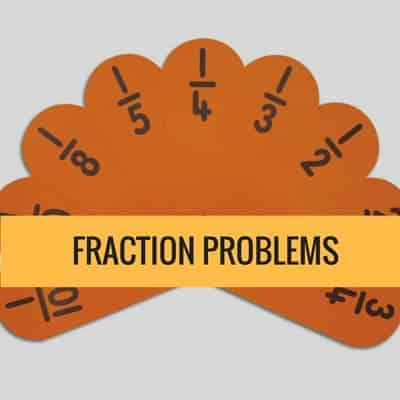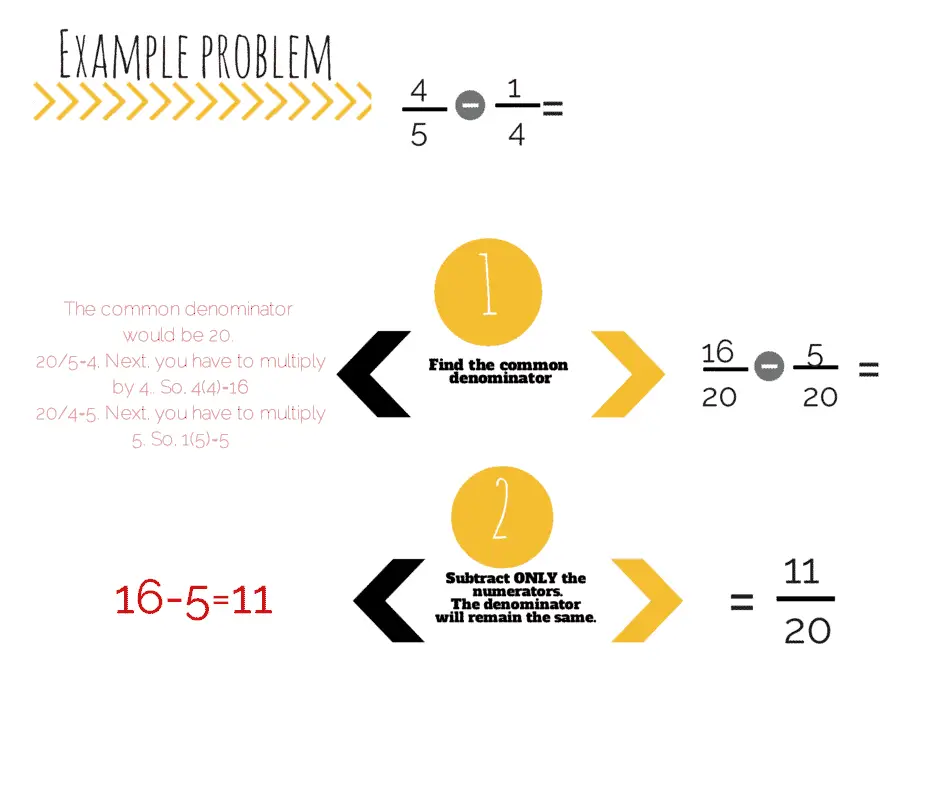# GED Math Practice Fraction Problems

### Fraction TutorialWe have GED Math Video Lessons

Check our Math Blueprint Video Course covering every possible topic for GED Math. It includes +100 videos, +2000 practice questions and loads of information.

### Practice Problems

Use these problems to practice fractions.
1. 15/187/9=

2. 3/5 + 5/15=

3. 3/4 + 4/7=

4. 9/153/5

5. 4/52/3

6. 1/5 x 2/3